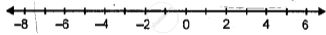# Fill in the Blanks, Using the Following Number Line: -6 is ______ than 3 and the Opposite of -6 is ______Than Opposite of 3. - Mathematics

Fill in the Blanks

Fill in the blanks, using the following number line:-6 is ______ than 3 and the opposite of -6 is ______than opposite of 3.

#### Solution

-6 is less than 3 and the opposite of -6 is greater than the opposite of 3.

Is there an error in this question or solution?

#### APPEARS IN

Selina Class 6 Mathematics
Chapter 7 Number Line
Exercise 7 (A) | Q 1.7